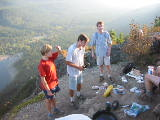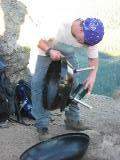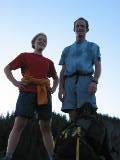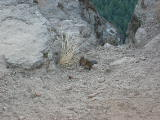## Rattlesnake Ledge TNAB Cookout

Each link with an 'a_' in the name is a link directly to a JPG file.The 'height=' links are to html pages that only contain the image and links to the previous and next image pages. A tag within the html file tells your browser to rescale the image to a given height. This is useful for viewing on smaller monitors.

 01a_Rattlesnake.jpgheight=480 height=600 height=750 02a_Rattlesnake.jpgheight=480 height=600 height=750 03a_Rattlesnake.jpgheight=480 height=600 height=750 04a_Rattlesnake.jpgheight=480 height=600 height=750 05a_Rattlesnake.jpgheight=480 height=600 height=750 06a_Rattlesnake.jpgheight=480 height=600 height=750 07a_Rattlesnake.jpgheight=480 height=600 height=750 08a_Rattlesnake.jpgheight=480 height=600 height=750 09a_Rattlesnake.jpgheight=480 height=600 height=750 10a_Rattlesnake.jpgheight=480 height=600 height=750 11a_Rattlesnake.jpgheight=480 height=600 height=750 12a_Rattlesnake.jpgheight=480 height=600 height=750 13a_Rattlesnake.jpgheight=480 height=600 height=750 14a_Rattlesnake.jpgheight=480 height=600 height=750 15a_Rattlesnake.jpgheight=480 height=600 height=750 16a_Rattlesnake.jpgheight=480 height=600 height=750